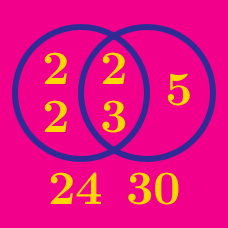Number Theory

# Greatest Common Divisor / Lowest Common Multiple: Level 4 Challenges

$\text{lcm}(1,77) + \text{lcm}(2,77) + \text{lcm}(3,77) + \cdots + \text{lcm}(77,77) = \, ?$

Notation: $\text{lcm}(a,b)$ denote the Lowest Common Multiple of $a$ and $b$.

For $m>1$, it can be proven that the integer sequence $f_m(n) = \gcd(n+m,mn+1)$ has a fundamental period $T_m.$ In other words, $\forall n \in \mathbb{N}, \space f_m(n+T_m) = f_m(n).$ Find an expression for $T_m$ in terms of $m,$ and then give your answer as $T_{12}.$

When 2017 is divided by a 2-digit number, what is the largest possible remainder?

Bonus: Generalize this problem.

Find the largest integer $n$ such that $n$ is divisible by all positive integers less than $\sqrt{n}$.

Find the sum of all integers $k$ with $1\leq k\leq 2015$ and $\gcd(k,2015)=1$.

What is the greatest common factor of all integers of the form $p^4 - 1$ where $p$ is a prime number greater than 5?

×